Dr. P.V. Viswanath
 Home Bio Courses Research Economics/Finance on the Web Student InterestCourses/Classnotes# Short sales and Margin Purchases

Investors can trade through a broker in one of two ways: they can either use their own money, or they can open a margin account with the broker. In this case, they use the broker's money, which he lends to them at a stipulated rate of interest. The advantage of borrowing the money in this manner from the broker is that the broker can lend to the trader at a lower rate of interest, since he has the securities in his possession and can use them as collateral.

Since the securities purchased by the trader function as collateral for the loan, the current market value of the loan is crucial vis-à-vis the amount lent. In order that the market value of the securities does not fall too low, the broker requires that the ratio of market value to the loan always exceed a certain proportion. Alternatively, the customer's equity in the securities as a proportion of the total value of the securities cannot fall below a specified proportion. This proportion is termed the margin requirement. Currently, the legal margin requirement on all stocks is 50%; i.e. at least 50% of the price of the securities should be financed by the customer himself. However, the broker can demand a higher margin level. This margin requirement at the time of initial purchase, which is termed the initial margin is larger than the margin requirement at a later time.

The initial margin requirement is given by the ratio:.

The actual margin at any time is given by: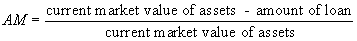. The minimum value of the AM that is permitted by the broker is called the maintenance margin (MM). Here's an example of how the maintenance margin comes into play.

Suppose, to begin with, the customer borrows 50% of the value of the stocks purchased. As the market value of the stocks drops, the actual margin will drop until, if the price drops enough, it will drop below the MM. As soon as this happens, the account is said to be undermargined. At this point, a margin call will be made by the broker, requiring the investor to add more liquid funds to his account, until the AM is greater than or equal to the MM. The normal practice among brokers is only to require enough additional funds for the AM to come up to the MM level. However, it is possible for the broker to require that, once the AM has dropped below the MM, it should be brought up to some higher margin level, such as the IM level.

On the other hand, if the market value of the assets rises, the account becomes overmargined. If the actual margin is greater than the IM, it is unrestricted, and the trader can withdraw the excess of the funds above the IM. If the AM is between the IM and the MM, no margin calls are made, but no funds can be withdrawn either. However, different brokerage houses have different rules, and some of them may permit cash withdrawals provided maintenance margin limits are met.  Conversely, other brokerage houses do not actually permit excess funds to be withdrawn in cash, but will allow them to be used for other transactions.  Finally, some brokerage houses will set aside any funds that exceed initial margin requirements, and will allow them to be withdrawn, even if subsequently actual margin drops below the initial margin level.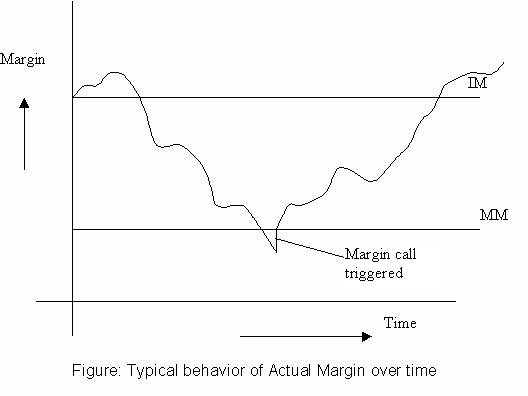Example 1:

Consider the following example: Martin buys 200 shares of MBIA stock at 32 3/4, on margin. The initial margin requirement is 50%. Hence he needs to put in 0.5(32.75 x 200) = \$3275 of his own money. At this point, the AM = IM. If the stock price should drop far enough, Martin would receive a margin call. How low can the price drop without triggering a margin call? Suppose the maintenance margin requirement is 35%. Then, the price could drop up to the value of P that satisfies the equation:

(200P - 3275)/(200P) = 0.35.

where the assets in the account equal 200P, and the amount of the loan is \$3275. Solving, we get a value of P = 25.19. Now suppose the price drops to \$22. What is the amount of the additional margin that is necessary for the AM to get back up to the MM level? This can be computed by solving the following equation:

(200 x 22 + Y -3275)/(200 x 22) = 0.35

Solving, we get \$415. At this point, the actual margin would be equal to (4400+415-3275)/(4400) = 0.35 as required.

Example 2:

Q. Bret Barakett has a margin account and deposits \$50,000. Assuming the prevailing initial margin requirement is 40%, commissions are ignored, and Reebok is selling at \$35 per share, how many shares can Brett purchase using the maximum allowable margin?

Solution: Brett can buy 50000/(.4)(35) or 3571.43 shares

Q. What is Brett's profit/loss if the price of Reebok stock falls to \$25?

Solution: If the price falls to \$25, the loss is (35-25)(3571.43) or 35714.30

Q. If the maintenance margin is 30%, to what price can Reebok fall before Brett will receive a margin call?

Solution: The price can fall up P, where P is given by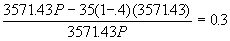, or P = \$30.

Q. How much additional money must Brett deposit if the stock price drops to \$5 below the level computed in c) above, and he is required to bring up the actual margin on his account to the maintenance margin?

Solution: If the price falls to \$25, he needs to have 0.3(25)(3571.43) or \$26785.73; he originally had \$50,000, of which he lost 35714.30. Hence he now needs an additional 26785.73 - (50000-35714.30) = \$12500.

Alternatively, the broker’s loan is now restricted to 70% of the value of the securities or .7(25)(3571.43) = 62500. The original loan amount was 75000. Hence the amount that the customer must pay up is 75000 - 62500 = \$12500.

Account Information:

At the beginning:

 Assets Liabilities Cash \$50000.00 Equity \$50000.00 Total \$50000.00 Total \$50000.00

Margin = 50000/50000 = 100%

After the shares are purchased:

 Assets Liabilities 3571.43 shares of Reebok at \$35 each. 125000.00 Loan 75000.00 Equity 50000.00 Total 125000.00 Total 125000.00

Margin = 50000/125000 = 40%

After the share price falls to \$25:

 Assets Liabilities 3571.43 shares of Reebok at \$25 each. 89285.71 Loan 75000.00 Equity 14285.71 Total 89285.71 Total 89285.71

Margin = 14285.71/89285.71 = 16%

After the margin call is paid:

 Assets Liabilities 3571.43 shares of Reebok at \$25 each. 89285.71 Loan (75000.00 - 12500) 62500.00 Equity 26785.71 Total 89285.71 Total 89285.71

Margin = 26785.71/89285.71 = 30%

### Short Sales

#### The Logistics of a Short Sale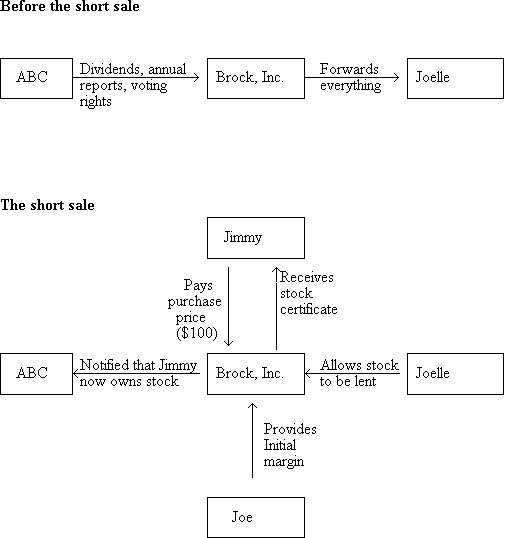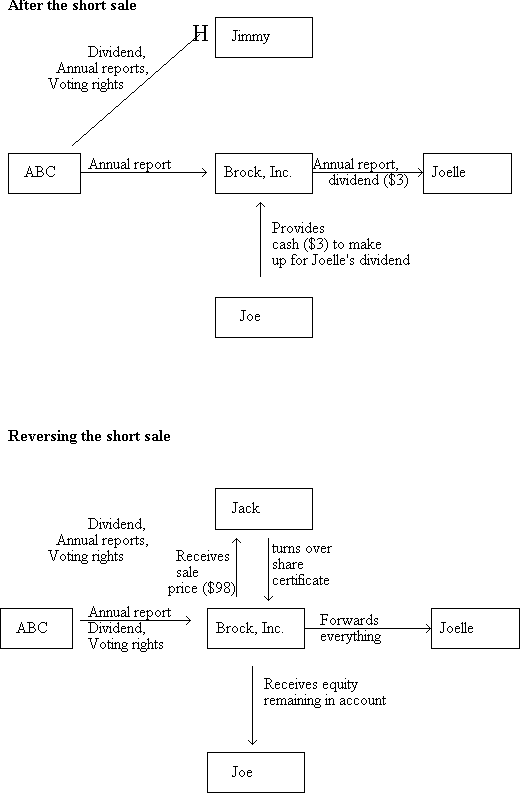#### Margin Computation with short sales:

Since a short seller of a stock has the corresponding obligation of buying back the stock at some point in the market, and returning it to the broker, the broker does not typically permit the shortseller to withdraw the proceeds of the short sale. In fact, he requires the shortseller to deposit a further sum of money in case the stock price rises, and the short seller should need additional funds to buy back the stock.

In the case of a short sale, the trader is borrowing, not cash, but rather securities, and margin rules apply here as well. The margin quantities, in this case is computed as follows: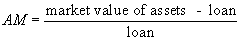, where the loan amount is the market value of the security borrowed at the time that the margin is being computed. Hence, if Tina sold short 200 shares of WMT (Wal-Mart Stores, Inc.), when the stock was selling at \$25, assuming a 50% initial margin, she would have had to put \$25 x 200 x 50% = \$2500 into her account., so that the loan of 200 x \$25 or \$5000 satisfies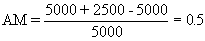, where the first term of \$5000 in the numerator refers to the original proceeds of the short sale of stock, the second term of \$2500 refers to the money that was put up as margin, and the third term of \$5000 refers to the value of the loan made to the trader. Just as in the case of a margin purchase, we have maintenance margin requirements here, as well. Suppose this is 25%. Then, if the market value of the stock rises to \$35, the actual margin, now, is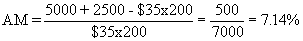. Since this is less than 25%, Tina must put up enough money to bring up the margin to the required initial margin of 50%, once again. This amount of money can be computed by solving the equation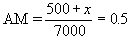, which works out to \$3000.

Normally, the broker will also allow the trader to use Treasury bills to meet margin calls since they are very liquid and essentially equivalent to cash. However, this depends on the agreement between the trader and the broker.

If the customer has purchased a stock on margin and short sold another stock, the total dollar margin required on the account is computed by aggregating the dollar amounts required for each separately. Thus, if we require a maintenance margin of 25% for both short sales and margin purchases, this means the following. For the margin purchase, we require, i.e. equity  must be greater than or equal to 0.25(current market value of assets). For the short sale, we require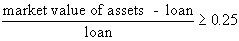, i.e. equity must be at least 25% of the loan amount. Hence if the current market value of securities purchased on margin is \$200 with \$165 borrowed from the broker, and if the current market value of securities shortsold is \$160, the total amount of equity that must be held in the customer's account is 0.25(200) + 0.25(160) = \$90.  If the shortsold securities were originally trading at \$130 at the time they were shortsold, the amount of cash realized from the short sale would have been 1.5(130) = \$195, assuming that initial margin was 50%; since the current market value of the shortsold securities is \$160, the amount of equity is 195 - 160 = \$35 on the short sale side.  On the margin purchase side, the amount of equity is 200 - 165 = 35, for a total equity of 35 + 35 = 70.  Hence, the difference of \$20 (90 - 70) must be deposited in cash.

### Example:

Jaeger Corporation stock currently sells for \$60 per share. The initial margin requirement is 50% and the maintenance margin is 30%. If Willie Danziger sells short 300 shares of Jaeger stock, to what price can the stock rise before Willie recieves a margin call?

Solution:

The formula for the actual margin is Percentage margin = Equity/Value of stock owed.

For our data, we get the equation 0.30 = (27000 - 300P)/300P, since at the beginning, Willie must have put up 300 x 60 x 0.5 in cash plus 300 x 60 from the original sale of the stock = \$27000. Solving our equation, we get P = \$69.23.

After the shares are short-sold:

 Assets Liabilities Cash from the sale of 300 shares at \$60 each = 18000 plus 9000 (300 x 60 x 0.5) put up additionally in cash 27000.00 Loan (equivalent to the current value of the shares borrowed on Danziger’s behalf, i.e. 300 x 60) 18000.00 Equity 9000.00 Total 27000.00 Total 27000.00

Margin = 9000/18000 = 50%

After the share price rises to \$69.23:

 Assets Liabilities Cash 27000.00 Loan (69.23 x 300) 20769.00 Equity 6231.00 Total 27000.00 Total 27000.00

Margin = 6231/20769 = 30%